Function Repository Resource:

# RatioSimplify

Simplify a list as if it were a ratio

Contributed by: Wolfram|Alpha Math Team
 ResourceFunction["RatioSimplify"][list] returns a simplification of list as a ratio in terms of integers, symbols and symbolic numbers. ResourceFunction["RatioSimplify"][list,type] returns the simplification of list as a ratio of the type type.

## Details and Options

ResourceFunction["RatioSimplify"] has the attribute HoldFirst.
ResourceFunction["RatioSimplify"] attempts to convert Real valued inputs to symbolic and Integer numbers.
Input type can be any of "SumNormalizedToOne", "EntriesNormalizedToOne", "PieChart", "LowestTerms" or All.
ResourceFunction["RatioSimplify"][list, All] returns an Association of all properties.
The argument type defaults to "LowestTerms".

## Examples

### Basic Examples (3)

Simplify a basic ratio:

 In:=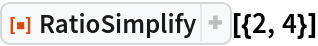Out=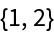Simplify a list with more elements according to the elements’ ratios:

 In:=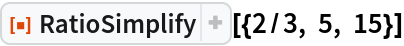Out=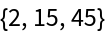Return all ratio simplification types as well as a PieChart visualizing the ratio:

 In:=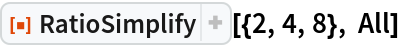Out=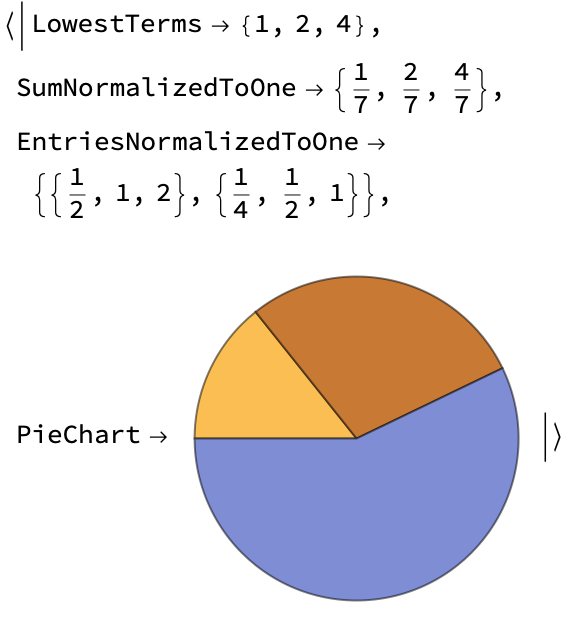### Scope (3)

Simplify a ratio that combines real-valued and exact-valued inputs:

 In:=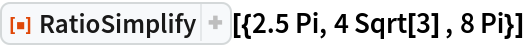Out=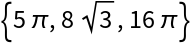Return the ratio with the sum of the entries normalized to 1:

 In:=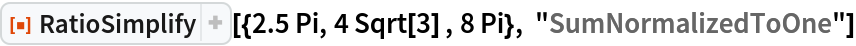Out=In:=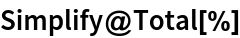Out=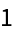RatioSimplify works with symbolic inputs as well as numeric:

 In:=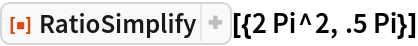Out=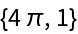In:=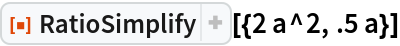Out=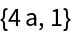### Possible Issues (2)

RatioSimplify returns unevaluated if it is unable to produce the specified result type:

 In:=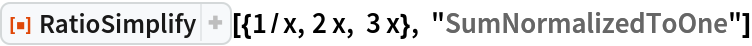Out=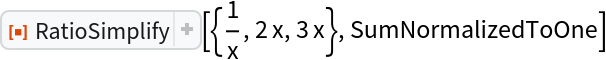Because RatioSimplify has the HoldFirst attribute, it may not evaluate to an expected result:

 In:=Out=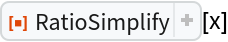In cases such as this, Evaluate can be used:

 In:=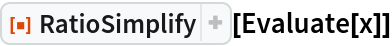Out=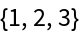## Publisher

Wolfram|Alpha Math Team

## Version History

• 2.0.0 – 23 March 2023
• 1.0.0 – 01 May 2020